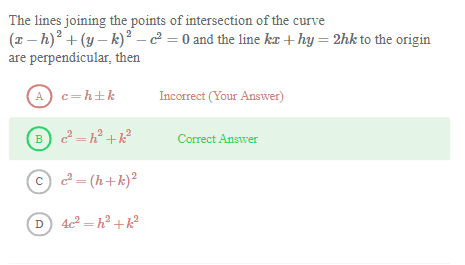# Points and linesalthough i did this for the second equation,
x/2h+y/2k=1
this represents an elipse
first equation is circle

#### Attachments

mfb
Mentor
I moved the thread to our homework section.
this represents an elipse
There are no squares in the equation. It is a straight line.

Did you draw a sketch?

•Suyash Singh
Mark44
Mentor
although i did this for the second equation,
x/2h+y/2k=1
Here's what you wrote:
$$\frac x 2 h + \frac y 2 k = 1$$

Here's what you meant:
$$\frac x {2h} + \frac y {2k} = 1$$
When you wrote those fractions on one line, the rules of PEMDAS dictate that the fractions x/2 and y/2 are multiplied by h and k, respectively. If you write them on one line, you need parentheses, like this x/(2h) + y/(2k) = 1.

As already noted, this equation is not the equation of an ellipse.

•Suyash Singh
epenguin
Homework Helper
Gold Member
Not all that easy question, especially as you have to read it half a dozen times to know what it actually is, but it's usually a help to know the answer, which apparently you do.

With that advantage you could think, hmm, Lines coming from points of intersection of a line with a circle that make a right angle – remember some well-known special case?
Can think if you make (x, y) = (0, 0) what happens to your equation to for the circle? That fits your case 2 and the circle going through the origin.
In which case right angle comes from lines from one point on the circumference to other two points on the cIrcumference.... again remind of anything?
Centre is the point (k, h).
Line from origin to centre would have slope h/k.
Line at right angles to that would have slope -k/h.
Would have equation kx + hy = something.
Ask if it goes through the centre - it all falls out.

Makes sense, you just have to be able to present it deductively starting from the question not the answer. Hope that helps

Last edited:
•Suyash Singh
Ray Vickson
Homework Helper
Dearly Missed
View attachment 225914
$$\frac{x}{2} h + \frac{y}{2} k = 1,$$
$$\frac{x^2}{h} + \frac{y^2}{k} = 1$$.
•